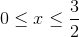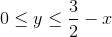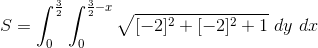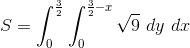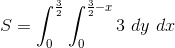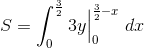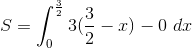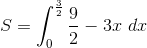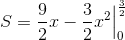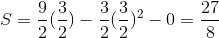# Calculus 3 : Surface Area

## Example Questions

### Example Question #1 : Surface Area

Find the surface area of the part of the plane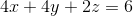in the first octant.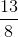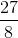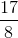Explanation:

Lets recall the equation of surface area.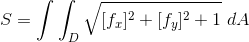Now we need to find all the neccessary equations to be able to evaluate the integral.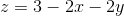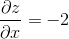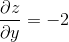We will plug in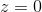, into the plane equation in order to get a line that intersects with the z axis.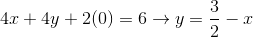Now we are going to set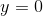, in the previous equation and solve for.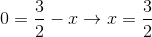We now have all the bounds for our double integral